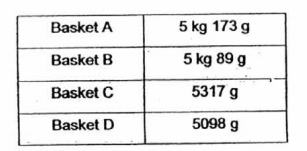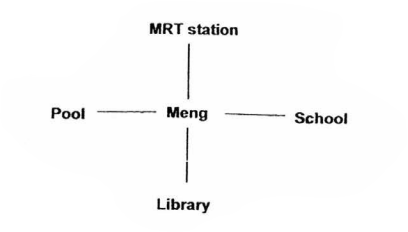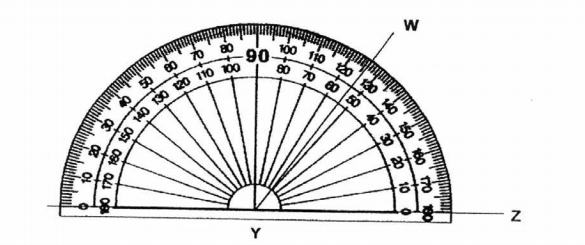# Primary 4 CA1 Practice #2

Time elapsed:
Q1. Which of the following numbers is the smallest number? 18 369, 18 639, 18 693, 18 936     [1 mark]
Q2. When a number is divided by 6, the quotient is 244 and the remainder is 5. What is the number?     [1 mark]
Q3. The table below shows the mass of four baskets. Which basket is the lightest?     [1 mark]Q4. Which of the following are factors of 36?     [1 mark]
Q5. Bruce bought 69 crates of fruits. In each crate, there were 17 papayas and some mangoes. He had a total 1242 mangoes. How many papayas and mangoes did he buy altogether?     [1 mark]
Q6. What is the remainder when 1928 is divided by 6?     [1 mark]
Q7. Round off 12 946 to the nearest ten.     [1 mark]
Q8. What is the sum of all the common factors of 15 and 30?     [1 mark]
Q9. Nathaniel wants to buy a storybook. The price of the storybook is divisible by 4, 6 and 8. What is the lowest possible price of the storybook?     [1 mark]
Q10. Ivan drove 8654 km on trip. Round off the distance travelled to the nearest ten.     [1 mark]
Q11. In 14 507, the digit '1' stands for _____.     [1 mark]
Q12. Meng is facing the library. Where will he be facing if he makes a 34 - turn in the clockwise direction?     [1 mark]Q13. Lucy packs 18 boxes of egg tarts. Each box contains 24 egg tarts. If there are 15 egg tarts left unpacked, how many egg tarts are there altogether?     [1 mark]
Q14. Which of the following statements is incorrect?     [1 mark]
Q15. 1304 x 7 = ____.     [1 mark]
Q16. What is 7595 when rounded to the nearest hundred?     [1 mark]
Q17. Which of the following is a multiple of both 6 and 8?     [2 marks]
Q18. Use the given protractor to find ∠WYZ.     [1 mark]Q19. In 18 053, the digit '5' stands for ___     [1 mark]
Q20. Which of the following has the greatest value?     [1 mark]